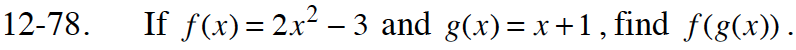Home > CCA2 > Chapter 12 > Lesson 12.1.4 > Problem12-78

12-78.

If f(x) = 2x2 − 3 and g(x) = x + 1, find f(g(x)). Homework Help ✎Substitute x + 1 into the equation for f(x),then simplify.

2x2 + 4x – 1